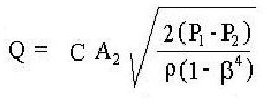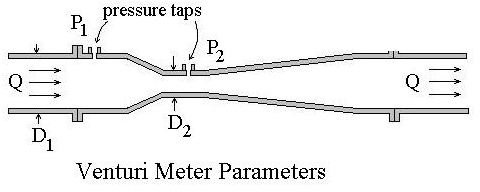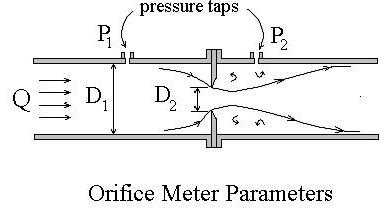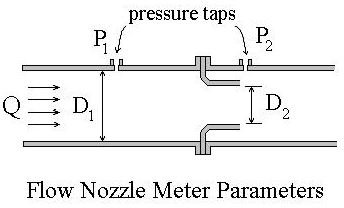# Pipe Flow Measurement with a Differential Pressure Flow Meter like the Orifice or Venturi Meter

## Understanding Pipe Flow Rate

The term pipe flow rate is often used to refer to flow rate for any closed conduit flow under pressure. The closed conduit is often circular, but may also be square or rectangular, such as a heating duct. The other major category of flow is open channel flow, which occurs when there is a free liquid surface open to atmospheric pressure. Pipe flow measurement is often done with a differential pressure flow meter like the orifice, flow nozzle, and ventruri meter, which are discussed in this article.

## The Differential Pressure Flow Meter

A general equation for a differential pressure flow meter is given at the right, where

Q = flow rate through pipe and meter, cfs,

C = discharge coefficient for the particular meter, dimensionless,A2 = constricted area perpendicular to flow, ft2,

P1 = undisturbed upstream pressure in the pipe, lb/ft2,

P2 = Pressure in the pipe at the constricted area, A2, lb/ft2,

β = D2/D1 = (diam. at A2/pipe diam.), dimensionless,

ρ = fluid density, slugs/ft3,

An Excel spreadsheet works well for differential flow meter calculations. For downloadable Excel templates to use for calculations with this equation, see the article, "Excel Templates for Venturi and Orifice Flow Meter Calculations."

## The Venturi MeterThe entrance to a venturi meter is a converging cone with a 15o to 20o angle. It converges down to the throat, which is the point of minimum cross-sectional area, maximum velocity, and minimum pressure in the meter. The exit portion of the meter is a diverging cone with an angle of 5o to 7o, which completes the transition back to full pipe diameter. The diagram at the left shows a typical venturi meter configuration with the parameters, D1, D2, P1 and P2 identified. Because of the smooth gradual transition down to the throat diameter and back to the full pipe diameter, the friction loss in a venturi meter is quite small. This leads to the value of a venturi meter discharge coefficient, C, being nearly one. Typical discharge coefficient values for a venturi meter range from 0.95 to as high as 0.995.

## The Orifice Flow Meter

The orifice meter is the simplest of the three types of differential flow metersbeing considered here. It is just a circular orifice plate with a hole in the middle, usually held in place between pipe flanges, as shown in the figure at the right. Because of the abrupt constriction at the orifice plate in an orifice meter, it has more frictional head loss than a venturi meter and a lower value for its discharge coefficient, C. A typical value for an orifice meter discharge coefficient is between 0.58 and 0.65.

## The Flow Nozzle MeterA flow nozzle meter consists of a short nozzle, usually held in place between two pipe flanges as shown in the diagram at the left.. It is simpler and less expensive than a venturi meter, but not as simple as an orifice meter. The frictional loss in a flow nozzle meter is much less than in an orifice meter, but higher than in a venturi meter. A typical flow nozzle discharge coefficient value is between 0.93 and 0.98.

## Conclusion

With any of these three types of meters for pipe flow measurement, a means is needed to measure the pressure difference across the meter, p1 – p2. Also the meter and pipe dimensions must be known and a value for the discharge coefficient is needed. The latter is typically available from the meter manufacturer.

## References and Image Credits

Images – drawn by H. Bengtson

References for further information:

1. Munson, B. R., Young, D. F., & Okiishi, T. H., Fundamentals of Fluid Mechanics, 4th Ed., New York: John Wiley and Sons, Inc, 2002.

2. U.S. Dept. of the Interior, Bureau of Reclamation, 2001 revised, 1997 third edition, Water Measurement Manual, available for on-line use or download at: https://www.usbr.gov/pmts/hydraulics_lab/pubs/wmm/index.htm

3. International Organization of Standards – ISO 5167-1:2003 Measurement of fluid flow by means of pressure differential devices, Part 1: Orifice plates, nozzles, and Venturi tubes inserted in circular cross-section conduits running full. Reference number: ISO 5167-1:2003.

## This post is part of the series: Measurement of Pipe Flow Rate

Measurement of pipe flow rate can use various flow meters, including a differential pressure flowmeter, like the orifice meter, venturi meter and flow nozzle meter. Other types of liquid flow meter are the rotameter, magnetic flow meter, ultrasonic meter, turbine flow meter and coriolis flow meter.